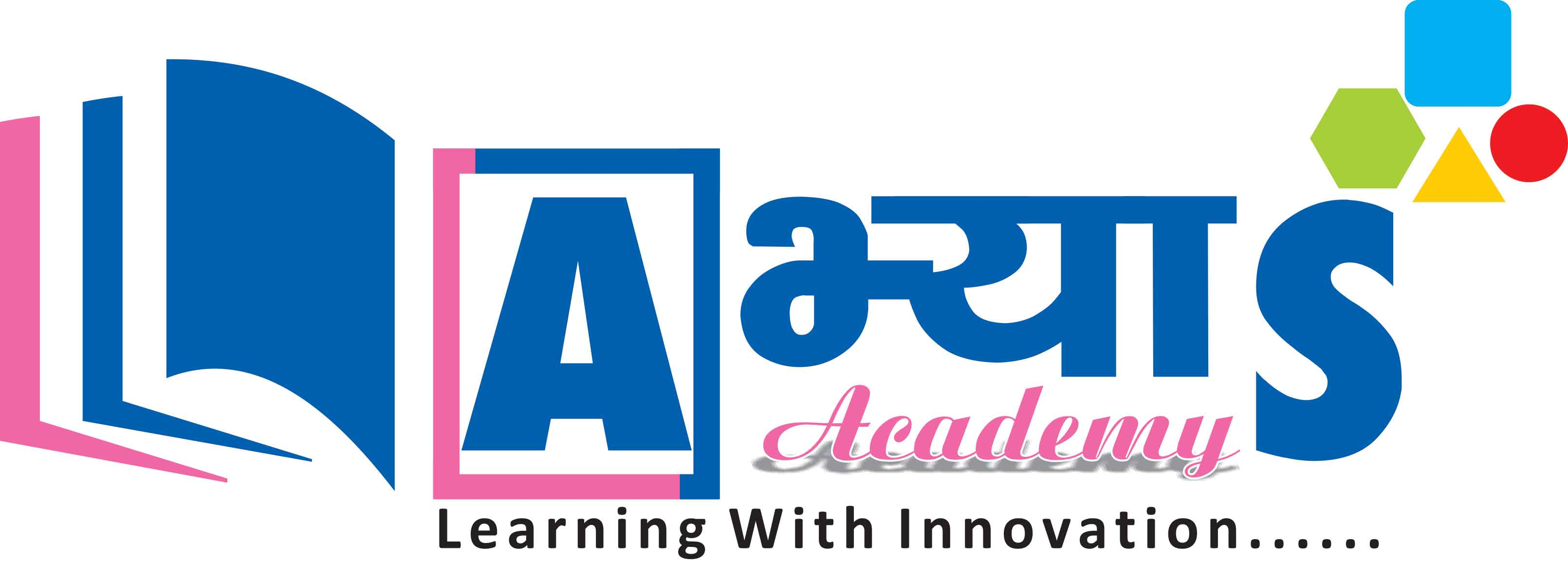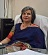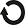Concept Detail
Maths / Mensuration / Area of Path and Verandah
(A Brief Glimpse of ABHYAS Content - Have aLook !!!!)

Area of Path and Verandah

Area of Path and Verandah:

It is observed that in square or rectangular gardens or parks. Some space in the form of path is left inside or outside or in between as cross paths.

Illustration: A footpath of uniform width 5 m runs round the inside of a rectangular park 38 m long and 32 m wide. Find the area of the path.

Solution: Let ABCD be the rectangular park and PQRS be the internal boundaries of the path.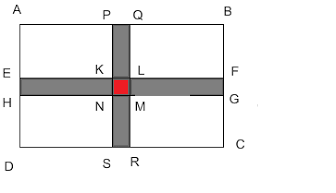We have, Length AB= 38 m and Breadth BC= 32 m:

Thus, Area of rectangle ABCD = AB  BC = 38 m  32 m = 1216

Now, Length PQ  = 38 m - 5 m - 5 m = 28 m and Breadth QR = 32 m - 5 m - 5 m = 22m

Area of rectangle PQRS = PQ  RS = 28 m  22 m = 616

Area of footpath = Area of rectangle ABCD - Area of rectangle PQRS = 1216 - 616=600

Thus, Area of footpath =600

The area of the crossroads = Area of the two colored rectangles - Area of the red colored rectangle at the centre.

We are subtracting the area of the rectangle because we are taking it into consideration two times.

Illustration: A rectangular lawn is 30 m by 20 m .It has two roads each 2 m wide running in the middle of it, one parallel to the length and the other parallel to the breadth . Find the area of the roads.

Solution: Let ABCD and PQRS be the cross roads,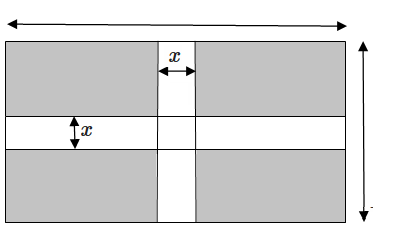we have AB = 2 m and BC = 30 m, then

Area of path ABCD =  AB  BC = 2 m    30 m  = 60

Now, for the other crossroad, PQ = 2 m and QR = 20 m,

then Area of path  PQRS = PQ   QR = 2 m    20 m = 40

Area EFGH is common to the both the paths: EF = 2 m and FG = 2 m, then Area of EFGH  =  EF  FG = 2 m   2 m = 4

Total area covered  as roads = Area of road ABCD + Area of road PQRS - Area of  EFGH = 60 + 40 - 4 =  100 - 4  = 96

Thus, total area of the roads = 96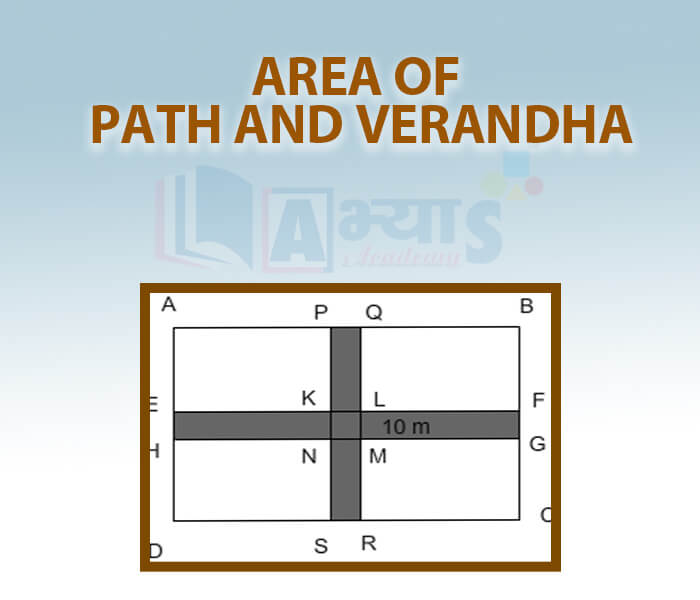A Brief Look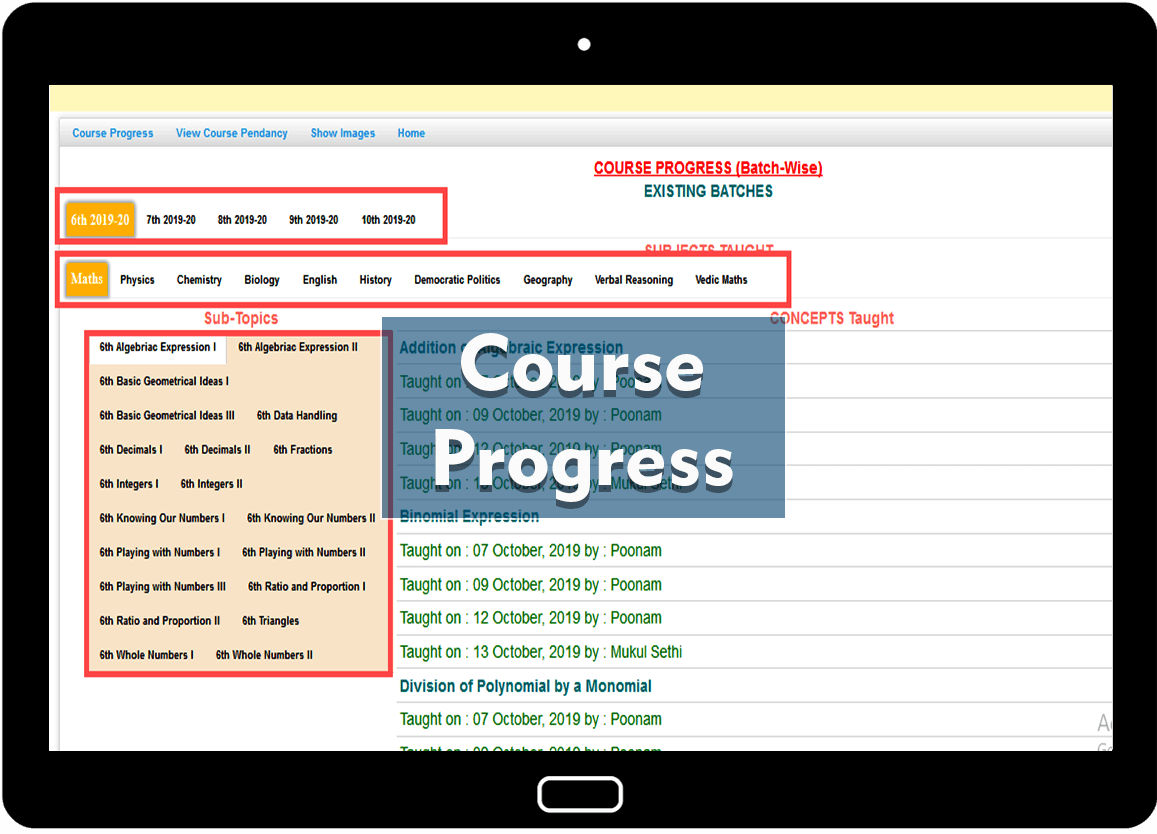Course Progress PANEL
Student can view their progress from this panel.
Self Study
Student can learn from Self Study panel. Concept notes and Video will appear their to learn concept. Exam will be held on the basis of concepts learn.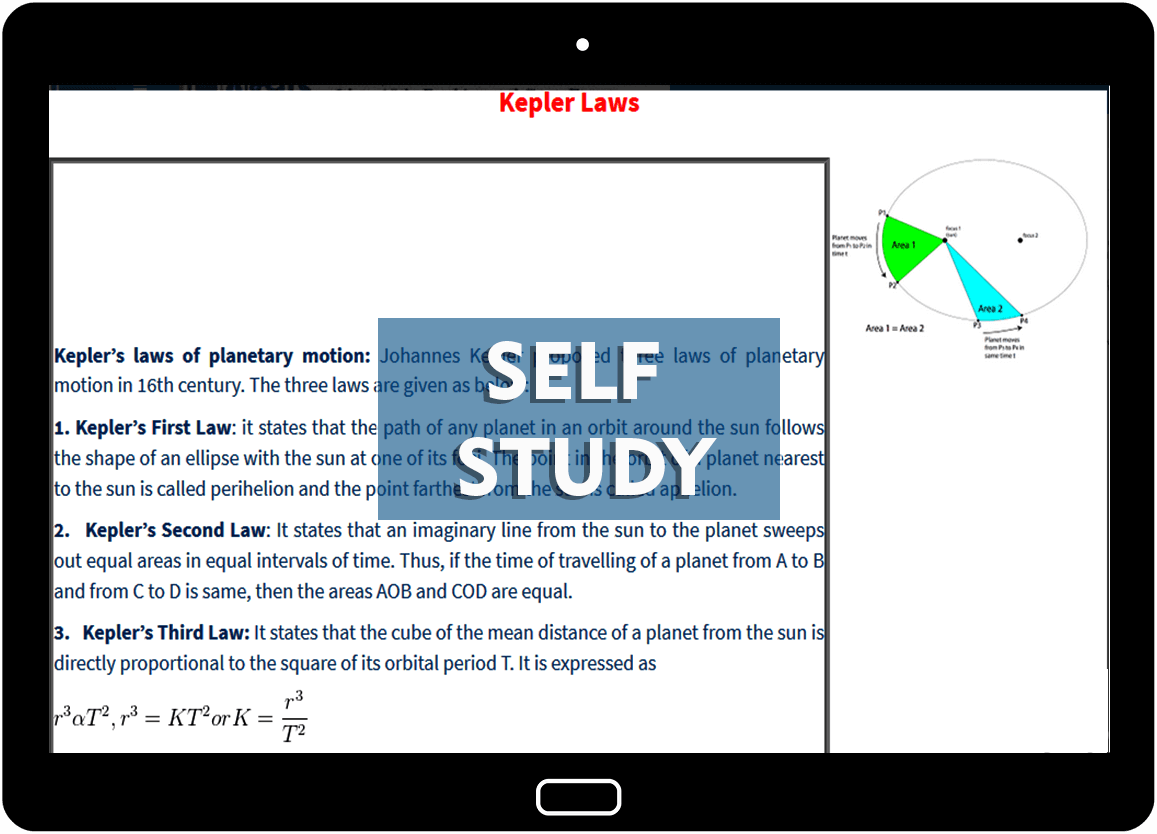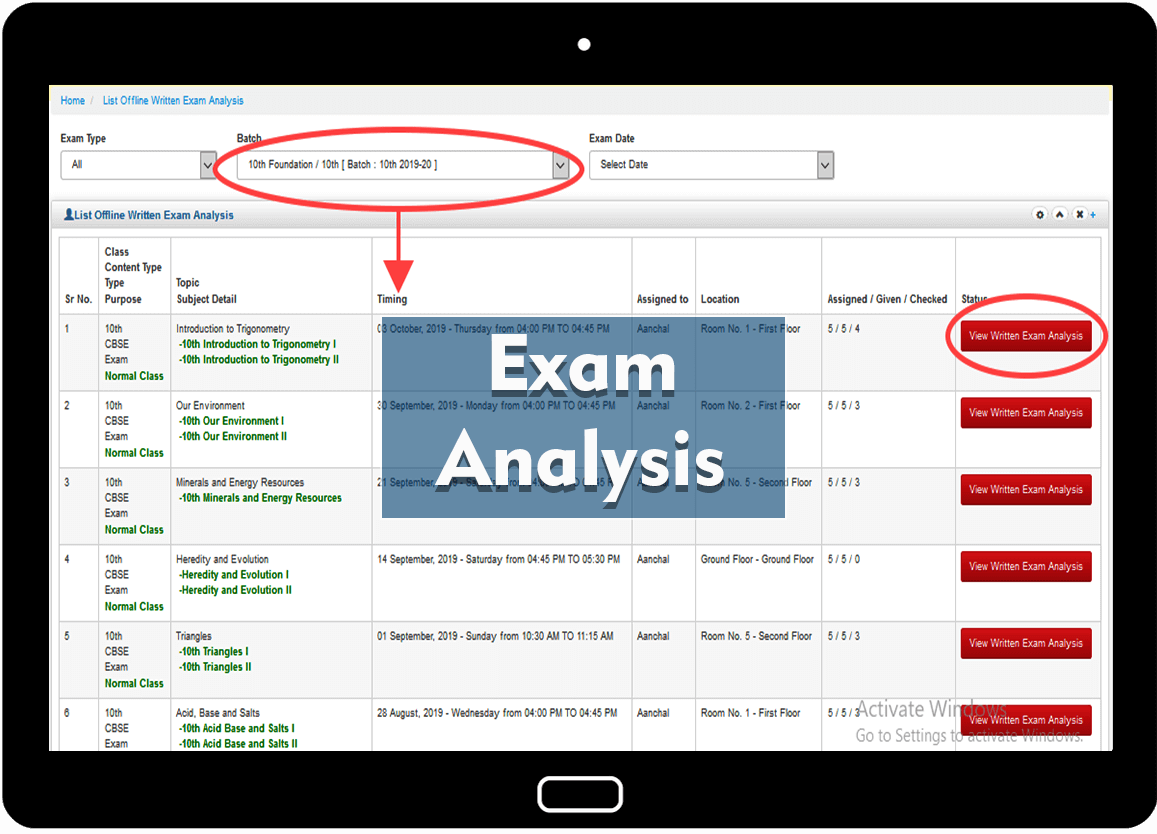Exam Evaluation PANEL
Detailed Exam Analysis for Offline and online exams will be Available in Student dashboard. Offline exams will be held for in-campus students only.
Class Notes PANEL
Student can view / download Class Notes assigned to them. Request can also be sent for elapsed Notes.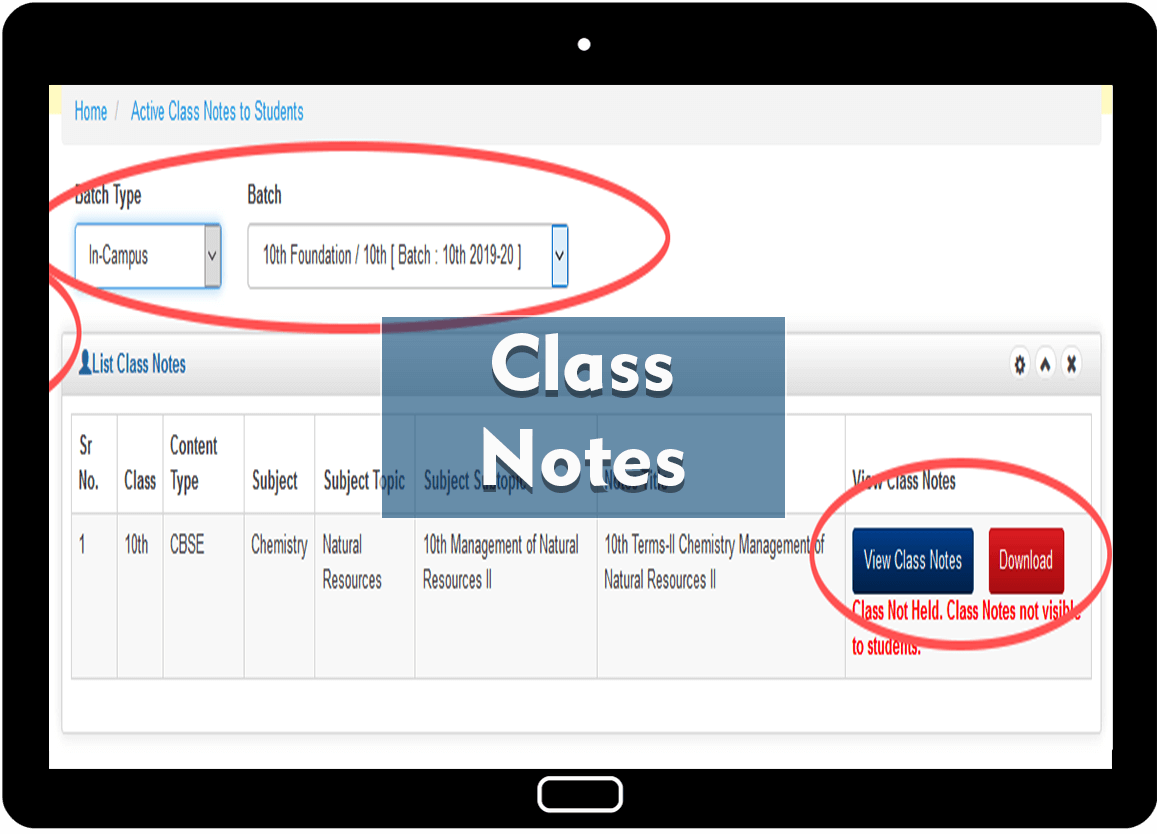REPORT CARD
Report Card of each student is visble in Student Panel for WISE-FA, WISE-PT, WISE-Testing, Written Exams, Attendance, etc.
STUDENT PANEL
Ranking amongst the students of the class are visible at any time. Students rank will automatically based on the exams given by the students and score.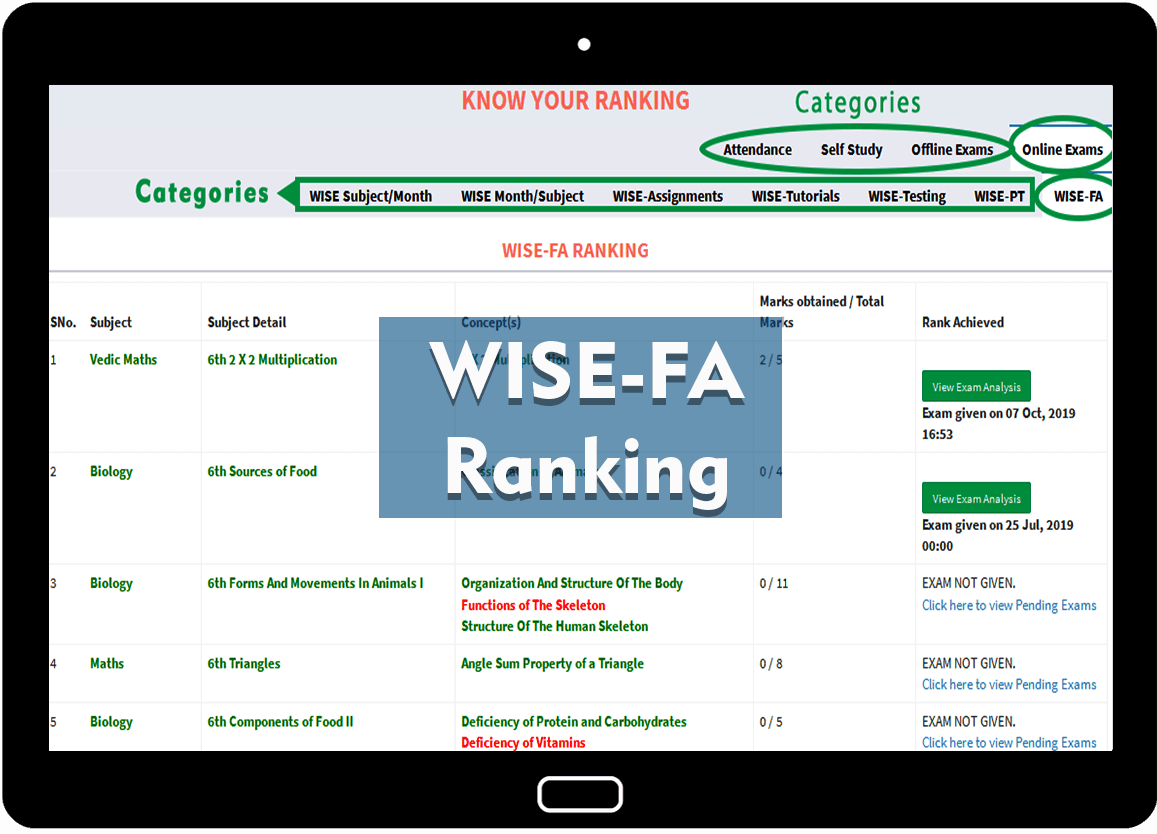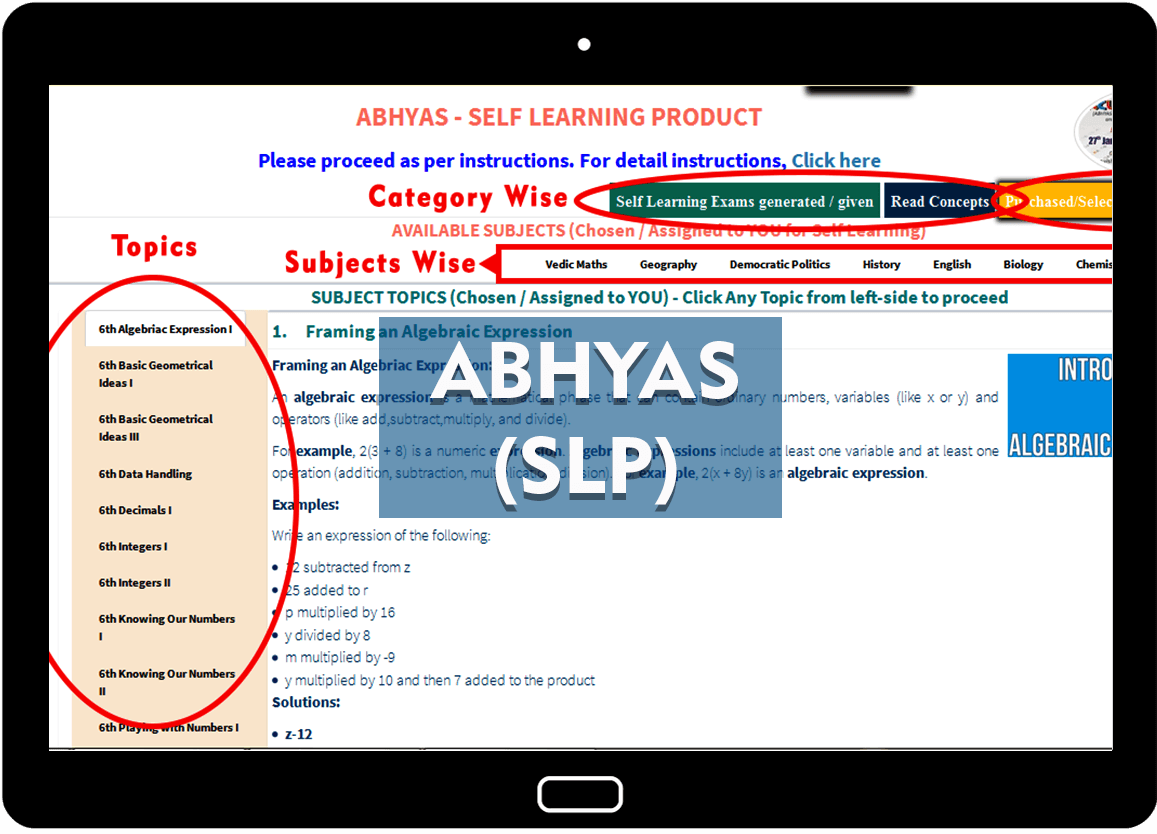SELF LEARNING PANEL
Self Learning panel to know topics/concepts read and exams given. Detail analysis provided to students for exams given. Remedial papers will be generated on student choice for Weak Concepts.
TEST GENERATOR
Test generator to generate test for concepts taught and pending.STUDENT DASHBOARD
To know Online and Offline exams given / pending with graphical view.